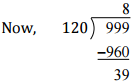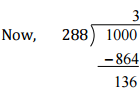Courses

# NCERT Solutions(Part - 3) - Playing with Numbers Class 6 Notes | EduRev

## Class 6 : NCERT Solutions(Part - 3) - Playing with Numbers Class 6 Notes | EduRev

The document NCERT Solutions(Part - 3) - Playing with Numbers Class 6 Notes | EduRev is a part of the Class 6 Course Mathematics (Maths) Class 6.
All you need of Class 6 at this link: Class 6

Exercise 3.6
Ques 1: Find the H.C.F. of the following numbers:
(a) 18, 48
(b) 30, 42
(c) 18, 60
(d) 27, 63
(e) 36, 84
(f) 34, 102
(g) 70, 105, 175
(h) 91, 112, 49
(i) 18, 54, 81
(j) 12, 45, 75
Ans: (a) Factors of 18 = 2 x 3 x 3
Factors of 48 = 2 x 2 x 2 x 2 x 3
H.C.F. (18, 48) = 2 x 3 = 6
(b) Factors of 30 = 2 x 3 x 5
Factors of 42 = 2 x 3 x 7
H.C.F. (30, 42) = 2 x 3 = 6
(c) Factors of 18 = 2 x 3 x 3
Factors of 60 = 2 x 2 x 3 x 5
H.C.F. (18, 60) = 2 x 3 = 6
(d) Factors of 27 = 3 x 3 x 3
Factors of 63 = 3 x 3 x 7
H.C.F. (27, 63) = 3 x 3 = 9
(e) Factors of 36 = 2 x 2 x 3 x 3
Factors of 84 = 2 x 2 x 3 x 7
H.C.F. (36, 84) = 2 x 2 x 3 = 12
(f) Factors of 34 = 2 x 17
Factors of 102 = 2 x 3 x 17
H.C.F. (34, 102) = 2 x 17 = 34
(g) Factors of 70 = 2 x 5 x 7
Factors of 105 = 3 x 5 x 7
Factors of 175 = 5 x 5 x 7
H.C.F. = 5 x 7 = 35
(h) Factors of 91 = 7 x 13
Factors of 112 = 2 x 2 x 2 x 2 x 7
Factors of 49 = 7 x 7
H.C.F. = 1 x 7 = 7
(i) Factors of 18 = 2 x 3 x 3
Factors of 54 = 2 x 3 x 3 x 3
Factors of 81 = 3 x 3 x 3 x 3
H.C.F. = 3 x 3 = 9
(j) Factors of 12 = 2 x 2 x 3
Factors of 45 = 3 x 3 x 5
Factors of 75 = 3 x 5 x 5
H.C.F. = 1 x 3 = 3

Ques 2: What is the H.C.F. of two consecutive:
(a) numbers?
(b) even numbers?
(c) odd numbers?
Ans: (a) H.C.F. of two consecutive numbers be 1.
(b) H.C.F. of two consecutive even numbers is 2.
(c) H.C.F. of two consecutive odd numbers is 1.

Que 3: H.C.F. of co-prime numbers 4 and 15 was found as follows by factorization:
4 = 2 x 2 and 15 = 3 x 5 since there is no common prime factor, so H.C.F. of 4 and 15 is 0.
Is the answer correct? If not, what is the correct H.C.F.?
Ans: No. The correct H.C.F. is 1.
Exercise 3.7
Ques 1: Renu purchases two bags of fertilizer of weights 75 kg and 69 kg. Find the maximum value of weight which can measure the weight of the fertilizer exact number of times.
Ans: For finding maximum weight, we have to find H.C.F. of 75 and 69.
Factors of 75 = 3 x 5 x 5
Factors of 69 = 3 x 69
H.C.F. = 3
Therefore the required weight is 3 kg.

Ques 2: Three boys step off together from the same spot. Their steps measure 63 cm, 70 cm and 77 cm respectively. What is the maximum distance each should cover so that all can cover the distance in complete steps?
Ans: For finding minimum distance, we have to find L.C.M of 63, 70 and 77.

 7 63, 70, 77 9 9,10,11 10 1, 10, 11 11 1, 1, 11 1, 1, 1

L.C.M. of 63, 70 and 77 = 7 x 9 x 10 x 11 = 6930 cm.
Therefore, the minimum distance is 6930 cm.

Ques 3: The length, breadth and height of a room are 825 cm, 675 cm and 450 cm respectively. Find the longest tape which can measure the three dimensions of the room exactly.
Ans: The measurement of longest tape = H.C.F. of 825 cm, 675 cm and 450 cm.
Factors of 825 = 3 x 5 x 5 x 11
Factors of 675 = 3 x 5 x 5 x 3 x 3
Factors of 450 = 2 x 3 x 3 x 5 x 5
H.C.F. = 3 x 5 x 5 = 75 cm
Therefore, the longest tape is 75 cm.

Ques 4: Determine the smallest 3-digit number which is exactly divisible by 6, 8 and 12.
Ans:

 2 6, 8,12 2 3, 4, 6 2 3, 2, 3 3 3, 1, 3 1, 1, 1

L.C.M. of 6, 8 and 12 = 2 x 2 x 2 x 3 = 24
The smallest 3-digit number = 100
To find the number, we have to divide 100 by 24
100 = 24 x 4 + 4
Therefore, the required number = 100 + (24 – 4) = 120.

Ques 5: Determine the largest 3-digit number which is exactly divisible by 8, 10 and 12.
Ans: L.C.M. of 8, 10, 12 = 2 x 2 x 2 x 3 x 5 = 120

 2 8, 10, 12 2 4, 5, 6 2 2, 5, 3 3 1, 5, 3 5 1, 5, 1 1, 1, 1

The largest three digit number = 999Therefore, the required number = 999 – 39 = 960

Ques 6: The traffic lights at three different road crossings change after every 48 seconds, 72 seconds and 108 seconds respectively. If they change simultaneously at 7 a.m. at what time will they change simultaneously again?
Ans: L.C.M. of 48, 72, 108 = 2 x 2 x 2 x 2 x 3 x 3 x 3 = 432 sec.
After 432 seconds, the lights change simultaneously.
432 second = 7 minutes 12 seconds
Therefore the time = 7 a.m. + 7 minutes 12 seconds
= 7:07:12 a.m.

 2 48, 72,108 2 24, 36, 54 2 12, 18, 27 2 6, 9, 27 3 3, 9, 27 3 1, 3, 9 3 1, 1, 3 1, 1, 1

Ques 7: Three tankers contain 403 litres and 465 litres of diesel respectively. Find the maximum capacity of a container that can measure the diesel of three containers exact number of times.
Ans: The maximum capacity of container = H.C.F. (403, 434, 465)
Factors of 403 = 13 x 31
Factors of 434 = 2 x 7 x 31
Factors of 465 = 3 x 5 x 31
H.C.F. = 31
Therefore, 31 litres of the container is required to measure the quantity.

Ques 8: Find the least number which when divided by 6, 15 and 18, leave remainder 5 in each case.
Ans: L.C.M. of 6, 15 and 18 = 2 x 3 x 3 x 5 = 90 Therefore, the required number = 90 + 5 = 95

 2 6, 15,18 3 3, 15, 9 3 1, 5, 3 5 1, 5, 1 1, 1, 1

Ques 9: Find the smallest 4-digit number which is divisible by 18, 24 and 32.
Ans: L.C.M. of 18, 24 and 32 = 2 x 2 x 2 x 2 x 2 x 3 x 3 = 288

 2 18, 24, 32 2 9, 12, 16 2 9, 6, 8 2 9, 3, 4 2 9, 3, 2 3 9, 3, 1 3 3, 1, 1 1, 1, 1

The smallest four-digit number = 1000Therefore, the required number is 1000 + (288 – 136) = 1152.

Ques 10: Find the L.C.M. of the following numbers:
(a) 9 and 4
(b) 12 and 5
(c) 6 and 5
(d) 15 and 4
Observe a common property in the obtained L.C.Ms. Is L.C.M. the product of two numbers in each case?
Ans: (a) L.C.M. of 9 and 4
= 2 x 2 x 3 x 3
= 36

 2 9,4 2 9,2 3 9,1 3 3,1 1,1

(b) L.C.M. of 12 and 5
= 2 x 2 x 3 x 5
= 60

 2 12,5 2 6, 5 3 3, 5 5 1,5 1,1

(c) L.C.M. of 6 and 5
= 2 x 3 x 5
= 30

 2 6, 5 3 3, 5 5 1,5 1,1

(d) L.C.M. of 15 and 4
= 2 x 2 x 3 x 5
= 60

 2 15,4 2 15,2 3 15,1 5 5,1 1,1

Yes, the L.C.M. is equal to the product of two numbers in each case. And L.C.M. is also the multiple of 3.

Ques 11: Find the L.C.M. of the following numbers in which one number is the factor of other:
(a) 5, 20
(b) 6, 18
(c) 12, 48
(d) 9, 45
What do you observe in the result obtained?
Ans: (a) L.C.M. of 5 and 20 = 2 x 2 x 5 = 20

 2 5, 20 2 5,10 5 5, 5 1,1

(b) L.C.M. of 6 and 18
= 2 x 3 x 3
= 18

 2 6, 18 3 3, 9 3 1, 3 1, 1

(c) L.C.M. of 12 and 48
= 2 x 2 x 2 x 2 x 3
= 48

 2 12,48 2 6, 24 2 3,12 2 3, 6 3 3, 3 1, 1

(d) L.C.M. of 9 and 45
= 3 x 3 x 5
= 45

 3 9, 45 3 3, 15 5 1, 5 1, 1

From these all cases, we can conclude that if the smallest number if the factor of the largest number, then the L.C.M. of these two numbers is equal to that of larger number.

Offer running on EduRev: Apply code STAYHOME200 to get INR 200 off on our premium plan EduRev Infinity!

## Mathematics (Maths) Class 6

185 videos|229 docs|43 tests

,

,

,

,

,

,

,

,

,

,

,

,

,

,

,

,

,

,

,

,

,

;